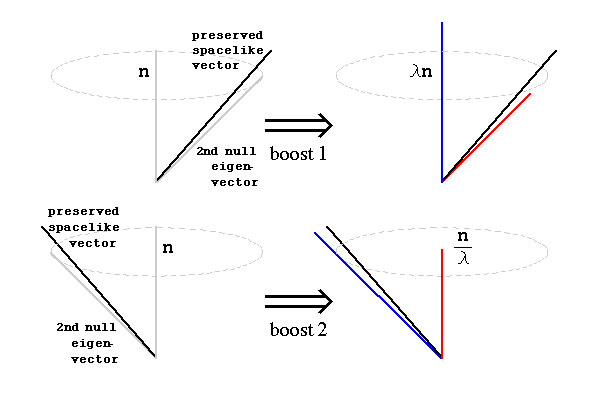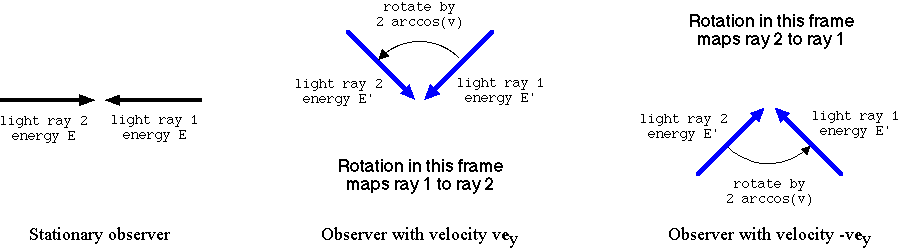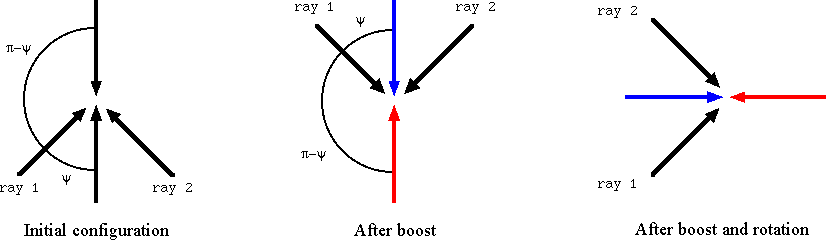# Null Rotations in 2+1 dimensions

## by Greg Egan

Null rotations are Lorentz transformations that preserve a null vector, just as ordinary rotations preserve a timelike vector and boosts preserve a spacelike vector.

## Five roads to null rotations

There are (at least) five ways to cook up a null rotation that preserves a given vector.

### 1. Exponentiate an element of the Lie algebra

One way to find a null rotation that preserves a given null vector n is to first find an element w of the Lie algebra so(2,1) that annihilates n. Exponentiating rw for some scalar r that quantifies the “amount of rotation” will then produce an element of SO(2,1) that preserves n.

For example, an element of so(2,1) that annihilates n = et + ex is:

 0 0 –1 0 0 –1 –1 1 0

If we call this w, then for any real number r, the matrix we get by computing exp(rw) is an SO(2,1) element that preserves n:

 1+r2/2 –r2/2 –r r2/2 1–r2/2 –r –r r 1

One question that immediately arises is, how do we know that we picked “the right” w? Any multiple of w, say sw, would also annihilate n, and if we used that instead we would have exp(r s w) as our family of null rotations.

The answer is that there’s no definitive choice for w; we are free to change the scaling as we wish. However, the next method shows that there is a natural map from vectors in R3 to elements of SO(2,1) where the scaling is set in a simple way by identifying each timelike vector with the rotation that preserves it and whose angle is equal to the vector’s length.

### 2. Take the limit of boosted ordinary rotations

Another way to obtain a null rotation that preserves the vector n is to consider an ordinary rotation R(θ) around some unit timelike vector et, use a boost to bring the axis of rotation closer to n, and take the limit as the boost goes to lightspeed.

Given a choice of et, we can always write n in the form:

n = r (et+en)

where en is a unit spacelike vector orthogonal to et, and r gives the size of the timelike and spacelike components of n in our chosen frame. We set:

L(θ,v) = B(v)–1R(θ)B(v)

where B(v) is a boost that takes the unit timelike vector:

e't = (et+ven)/√(1–v2)

to et. Then the vector:

p(v) = r √(1–v2) e't = r (et+ven)

will be preserved by L(θ,v). For all v<1, p(v) will be timelike, but it will tend towards n as v→1. The angle of the ordinary rotation is set to the length of p(v):

θ = √(–p(vp(v)) = r√(1–v2)

This angle tends to zero as v→1 and p(v) approaches n. We take the limit:

N(n) = limv→1L(r√(1–v2),v)

to obtain a null rotation N(n) that preserves n.

Note that although we needed to pick a basis in order to carry out this construction, the final result, N(n), is a basis-independent map from null vectors to linear transformations. If we picked a different basis to describe the same null vector n we would get a different component matrix for N(n), but it would still be the same linear transformation.

If we put n = r (et + ex), the matrix we obtain for N(n) by this method agrees with the result for method 1. Here, though, the overall scale is set by the fact that we chose to associate each timelike vector p(v) with a rotation by an angle equal to its length.

### 3. Multiply two boosts with a common null eigenvector

A third way to construct a null rotation is as the product of two boosts. Every boost, B, has two null eigenvectors, and their eigenvalues are reciprocals of each other; if the boost represents a change in speed of v, the eigenvalues are λ = √[(1+v)/(1–v)] and 1/λ = √[(1–v)/(1+v)]. In 2+1 dimensions there are precisely two null rays orthogonal to any given spacelike vector, and each of the null rays orthogonal to the spacelike vector preserved by B will contain one of B’s null eigenvectors. If two boosts, B1 and B2, have n as a common eigenvector, and the product of the eigenvalues is 1, then the product B1B2 will be a null rotation that preserves n.To give an example, two boosts that preserve the spacelike vectors et + ex + ey and et + exey respectively will both have n = et + ex as a null eigenvector, since n is a null vector orthogonal to both preserved vectors. If the boosts are of equal size and appropriately oriented their product will preserve n; “appropriately oriented” means one boost multiplies n by λ = √[(1+v)/(1–v)] and the other multiplies it by the reciprocal. The easiest way to construct each of these boosts explicitly is to start with the diagonal form and then apply a change of basis such that the appropriate eigenvalues are applied to the common null eigenvector, the preserved spacelike vector, and a second null eigenvector orthogonal to the preserved vector.

### 4. Multiply two ordinary rotations around different timelike vectors

If we choose a reference frame to work in, then imagine an observer moving relative to that frame, the observer will see the familiar effects of Doppler shift and aberration. If the observer is facing in the direction she is moving, a ray of light that would have been incident directly from the right if she had been stationary will appear to arrive at an angle of less than 90 degrees; to be precise, the angle will be equal to arccos(v), where v is the speed of the observer.

If the observer subjects that light ray to a counter-clockwise rotation of 2 arccos(v) in her own frame, its spatial component will be reflected in the line of motion, and it will become identical to a ray that would be seen as incident directly from the left by a stationary observer. Because the Doppler shift is symmetrical about the line of motion, the energy as well as the direction will match. In other words, a stationary observer would see the rotated ray as having its spatial component reversed and its time component unchanged.

For an observer moving with the same speed in the opposite direction, facing in his own direction of motion, exactly the same argument applies, except that this second observer will have opposite left/right labels for the same rays. So if he takes the ray that the first observer has rotated and applies his own rotation of 2 arccos(v), the light ray will end up restored to precisely its initial direction, and as already noted its time component will be unchanged. Thus the product of these two ordinary rotations preserves the null vector that describes the incident light ray.This scenario is easily generalised by allowing the two observers to have different speeds, v1 and v2, and to perform rotations in their respective frames of 2 arccos(v1) and 2 arccos(v2). Any situation where the product of two ordinary rotations is a null rotation can be described in this way, by a suitable choice of v1 and v2.

### 5. Multiply a boost by an ordinary rotation

The Doppler shift for light rays incident on a moving observer ranges from an increase in frequency for light from straight ahead through to a decrease for light from directly behind, so there is always a certain intermediate angle at which there is no Doppler shift. However, the light incident at this angle will be subject to aberration: a change in its spatial direction as measured by the moving observer compared to a stationary one. So a boost followed by an ordinary rotation, to compensate for the aberration experienced by those rays that undergo no Doppler shift, will produce a null rotation.

Specifically, a ray whose angle of incidence (measured in the stationary frame) from the direction of the observer’s motion is π–ψ will experience no Doppler shift, where ψ = arccos(√[(1–s)/(1+s)]) and s = √(1–v2). However, aberration causes the moving observer to see the ray as arriving at an angle of ψ. So a rotation of π–2ψ = arccos[(3s–1)/(s+1)] following the boost will yield a null rotation (assuming the ray arrives from the observer’s left; a rotation of π+2ψ will correct the aberration for the ray with no Doppler shift arriving from the right).Those angles are correct if the rotation takes place in our chosen “stationary” frame, around a time axis orthogonal to b, the spacelike vector preserved by the boost. We can generalise the situation by allowing the rotation to be around any timelike vector u, and adjusting the angle of rotation accordingly. The time axis of the stationary frame is then chosen to be coplanar with u and b and orthogonal to b, ensuring that the situation has the necessary symmetry to allow a rotation around u to compensate for the aberration. The vector u can then be thought of as the time axis of a second observer moving with a speed v2 in a perpendicular direction to the observer we associated with the boost, and the adjustment to the angle of rotation can be found by applying the formula for aberration relevant to that motion.

## Properties of null rotations

To make things concrete, we will set n = et + ex, in which case the null rotations that preserve n take the form:

 1+r2/2 –r2/2 –r r2/2 1–r2/2 –r –r r 1

Note that the trace of this matrix is a constant, 3, independent of r, so unlike the case with ordinary rotations (where the trace is 1+2cos(θ)), the trace cannot be used to identify the “amount of rotation”. In fact, the quantity r has no invariant meaning; it is basis-dependent, just as the individual components of a null vector are basis-dependent. If we switched to a reference frame that was moving in the ex direction, the transformed version of the matrix above would take the same general form but the value of r for any particular null rotation would be different. Other changes of reference frame would give matrices that are not of this form. A suitable choice of basis can always put a null rotation into this form, but the choice will not be unique.

Although it’s not hard to see that the matrix above will preserve n = et + ex, it’s easier to understand the action on other vectors if we switch to a new basis: {ey, n, n + ex}. This is not an orthonormal basis: n is a null vector, and the last two entries are not orthogonal. In this basis, the matrix becomes:

 1 0 r –r 1 –r2/2 0 0 1

If we call the three new coordinates y, n and z, then the plane z=0 is a plane tangent to the light cone; it contains n, and is also orthogonal to n. The other planes z=constant consist of points x that satisfy the equation x·n=z. The effect of the null rotation is to map (y,n,z) to (y+z r, nr yz r2/2, z). In other words, the planes z=constant are preserved as sets, and the coordinates within the plane, (y,n), are acted on by a shear transformation that subtracts r y from n, plus a translation by (z r, –z r2/2).

With ordinary rotations and boosts that preserve a vector a, the action of the Lorentz transformation on all of the affine planes x·a=constant is identical, and corresponds to identifying each plane with the plane x·a=0 via a projection. With null rotations, although the shear that is applied to each plane z=constant is the same, the translation by (z r, –z r2/2) makes it impossible to perform a similar identification.

Now, the squared magnitude of a vector x whose coordinates are (y,n,z) is:

x·x = y2 + 2 z n + 3 z2

so surfaces of constant magnitude intersect these planes along parabolas, except in the case z=0, when they are straight lines y=constant. The transformation (y,n,z) → (y+z r, nr yz r2/2, z) moves points along these parabolas; in the plane z=0 this becomes a pure shear transformation that moves points along lines of constant y.

The movie below shows the action on the plane z=0, the plane z=1/2, and in a region of spacetime around the origin. The colours red, black, and blue indicate timelike, null, and spacelike vectors.In the plane z=0, which consists of vectors orthogonal to n, the dot product of any vector with itself is y2, and the dot product of any vector with its image under the null rotation is also y2. So the dot product of a unit vector with its image, which for a normal rotation would equal the cosine of the angle of rotation, is 1, implying a rotation angle of zero. Of course this does not mean that the null rotation acts as the identity on the plane z=0; rather, the result arises because the induced metric on this plane is degenerate.Science Notes / Null Rotations in 2+1 dimensions / created Wednesday, 7 June 2006 / revised Wednesday, 5 July 2006